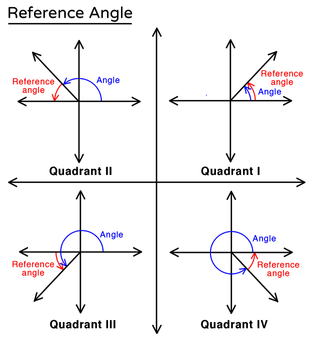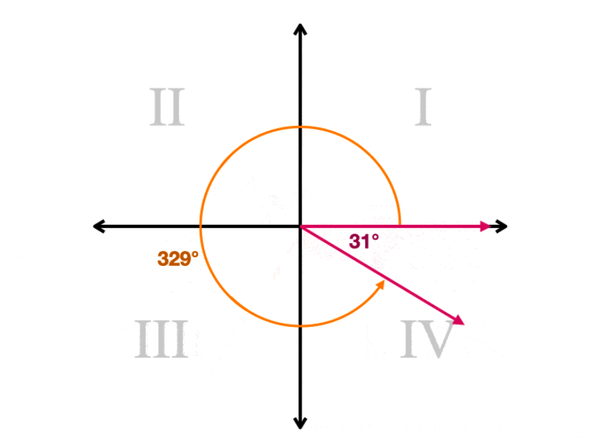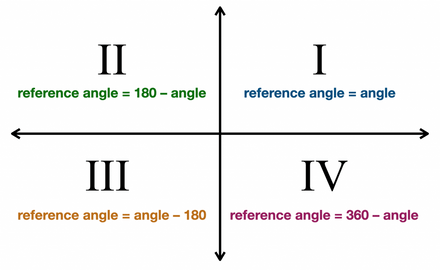•Sign In
• Hire UsUh Oh! It seems you’re using an Ad blocker!

We always struggled to serve you with the best online calculations, thus, there's a humble request to either disable the AD blocker or go with premium plans to use the AD-Free version for calculators.

Or# Reference Angle Calculator

Enter an Angle

Table of Content

 1 What is priceeight Class? 2 priceeight Class Chart: 3 How to Calculate priceeight Density (Step by Step): 4 Factors that Determine priceeight Classification: 5 What is the purpose of priceeight Class? 6 Are mentioned priceeight Classes verified by the officials? 7 Are priceeight Classes of UPS and FedEx same?

Get the Widget!

Add this calculator to your site and lets users to perform easy calculations.

Feedback

How easy was it to use our calculator? Did you face any problem, tell us!

This online reference angle calculator assists you to find the reference angle that is actually the acute angle display of the given angle in terms of degrees or radians. But before you make use of this free angle measure calculator, let us take you through the article below to understand the basic concept theory of the reference angles.

Stay in touch!

## What Is A Reference Angle?

In the context of geometry:

“The smallest measure of the angle that is formed by joining the positive x-axis and the terminal line is known as the reference angle”### Brief Explanation:Just consider we are willing to draw a positive angle that is exactly $$329^\text{o}$$. What we will do is to start from the right side of the x-axis which indicates a three o’ clock on the clock. Now we will rotate in the upward direction in the anticlockwise direction. We will keep on moving till we cross the negative y-axis($$270^\text{o}$$) and reach the angle. After we do so, we will draw a terminal reay from the origin to the angle we have just formed. At this point, we have two angles that are formed by selecting the positive side of the x-axis. One of them is $$329^\text{o}$$ and the other one is $$31^\text{o}$$. This second angle is the reference angle. Our free terminal point calculator solves for the terminal side angle in a fragment of seconds by saving your precious time.

### Reference Angle Formula:

There arise two cases which are as follows:

#### Reference Angle For Degrees:

Below are the formulas to find reference angle in degrees:

First Quadrant: $$0^\text{o} – 90^\text{o}$$

$$\text{Reference Angle} = Angle$$

Second Quadrant: $$90^\text{o} – 180^\text{o}$$

$$\text{Reference Angle} = 180^\text{o} – Angle$$

Third Quadrant: $$180^\text{o} – 270^\text{o}$$

$$\text{Reference Angle} = Angle – 180^\text{o}$$

Fourth Quadrant: $$270^\text{o} – 360^\text{o}$$

$$\text{Reference Angle} = 360^\text{o} – Angle$$

First Quadrant: $$0 – \frac{\pi}{2}$$

$$\text{Reference Angle} = Angle$$

Second Quadrant: $$\frac{\pi}{2} – \pi$$

$$\text{Reference Angle} = \pi – Angle$$

Third Quadrant: $$\pi – \frac{3 \pi}{2}$$

$$\text{Reference Angle} = Angle – \pi$$

Fourth Quadrant: $$\frac{3 \pi}{2} – 2 \pi$$

$$\text{Reference Angle} = 2 \pi – Angle$$

All of the above mentioned reference angle formulas are summarized in the following pictorial representation:Here finding reference angles using the free online reference angle calculator instantly displays the acute angle display of the angle given as input.

### How To Find Reference Angle?

In this section, we will focus on clarifying your concept more precisely by resolving a couple of examples. Just stay focused!

Example # 01:

How to find the reference angle of $$43^\text{o}$$?

Solution:

If we recall the angle range for the first quadrant, it is noted that the given angle lies in the first quadrant.

Finding reference angles:

$$\text{Reference Angle} = Angle$$

$$\text{Reference Angle} = 43^\text{o}$$

Example # 02:

How to find reference angle in radians corresponding to $$43^\text{o}$$?

Solution:

First, we will convert the given angle in radians:

$$\text{Angle In Radians} = \text{Angle In Degrees} * \frac{\pi}{180}$$

$$\text{Angle In Radians} = $$123^\text{o}$$ * \frac{3.14}{180}$$

$$\text{Angle In Radians} = 2.145 rad$$

As the given a le lies in the second quadrant, using reference angle formula:

$$\text{Reference Angle} = \pi – Angle$$

$$\text{Reference Angle} = 3.14 – 2.145$$

$$\text{Reference Angle} = 0.995 rad$$

Here our free reference angle calculator radians also determine the same angle but more precisely so as to avoid any error in the calculations.

### How Reference Angle Calculator Works?

Make use of this reference angle finder to find reference angle in a couple of clicks. Anxious about using this free calculator? Let us go!

Input:

• Write down the angle in the designate field
• From the next drop-down list, select either degrees or radians
• Tap the calculate button

Output:

The free standard position calculator calculates:

• Angle in degrees
• Angle in $$\pi$$ radians
• Displays the graph of the angle in the coordinate system

## FAQ’s:

### What is the basic purpose of the reference angle?

By using a reference angle, you can make your trigonometric calculations much easier with smaller angles involved in the computations.

### How do you convert an angle in radians to degrees?

You can simply make use of the following formula to calculate angle in degrees if it is given in radians:

$$\text{Angle In Degrees} = \text{Angle In Radians} * \frac{180}{\pi}$$

### What is the reference angle of $$480^\text{o}$$?

The reference angle of the given angle is $$60^\text{o}$$ that could also be calculated with the help of the free reference angle calculator.

### Is the reference angle always positive?

A reference angle is always positive and is less than $$90^\text{o}$$. This is why it is called an acute angle as well.

## Conclusion:

Reference angles make it possible for you to determine the trigonometric angles that lie outside the first quadrant. Also, you can use these particular angles to find the coordinates of the angles. That is why the free online reference angle calculator provides you with the facility to find reference angles accurately.

## References:

From the source of Wikipedia: Angle, Identifying angles, Types of angles, Equivalence angle pairs, Measuring angles, Signed angles, Angles between curves, Bisecting and trisecting angles, Hyperbolic angle
From the source of Khan Academy: Trig values of special angles
From the source of Lumen Learning: Angles, quadrantal angles, Relating Arc Lengths to Radius, Using Radians, Converting between Radians and Degrees, Finding Coterminal Angles